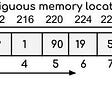# Build Array from Permutation — Day 103(Python)

Today, we will look into another easy problem.

1920. Build Array from Permutation

Given a zero-based permutation `nums` (0-indexed), build an array `ans` of the same length where `ans[i] = nums[nums[i]]` for each `0 <= i < nums.length` and return it.

A zero-based permutation `nums` is an array of distinct integers from `0` to `nums.length - 1` (inclusive).

Example 1:

`Input: nums = [0,2,1,5,3,4]Output: [0,1,2,4,5,3]Explanation: The array ans is built as follows: ans = [nums[nums], nums[nums], nums[nums], nums[nums], nums[nums], nums[nums]]    = [nums, nums, nums, nums, nums, nums]    = [0,1,2,4,5,3]`

Example 2:

`Input: nums = [5,0,1,2,3,4]Output: [4,5,0,1,2,3]Explanation: The array ans is built as follows:ans = [nums[nums], nums[nums], nums[nums], nums[nums], nums[nums], nums[nums]]    = [nums, nums, nums, nums, nums, nums]    = [4,5,0,1,2,3]`

Constraints:

• `1 <= nums.length <= 1000`
• `0 <= nums[i] < nums.length`
• The elements in `nums` are distinct.

Follow-up: Can you solve it without using an extra space (i.e., `O(1)` memory)?

We are given a zero-based permutation, which means in our array we have numbers from 0 to length(nums)-1. We need to return an array whose elements are positioned as nums[nums[i]].

`class Solution:    def buildArray(self, nums: List[int]) -> List[int]:        output = []        for n in nums:            output.append(nums[n])        return output`

Complexity

Time Complexity

Since we are just traversing through the array once, the time complexity is O(N).

Space Complexity

We are using extra space to store our result, hence space complexity is O(N).

Let us try to solve the follow-up question.

`class Solution:    def buildArray(self, nums: List[int]) -> List[int]:        return( nums[nums[i]] for i in range(len(nums)))`

Here we are running for-loop inside the return statement itself.

Time Complexity

Since we are just traversing through the array once, the time complexity is O(N).

Space Complexity

We are not using any extra space, and hence the space complexity will be O(1).

--

--

--

## More from Annamariya Tharayil

Software Engineer. Find me @ www.linkedin.com/in/annamariya-jt

Love podcasts or audiobooks? Learn on the go with our new app.

## How to Use Terraform with GKE: a Step-by-Step Guide to Deploying Your First Cluster## Introducing Chaos Platform 2.0 for Azure## How to Build a Car Racing Game in AR in 15 Minutes or Less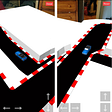## I’m Officially Vim User Now!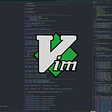## Create Custom Templates and Pages for WordPress## Creating Enemy AI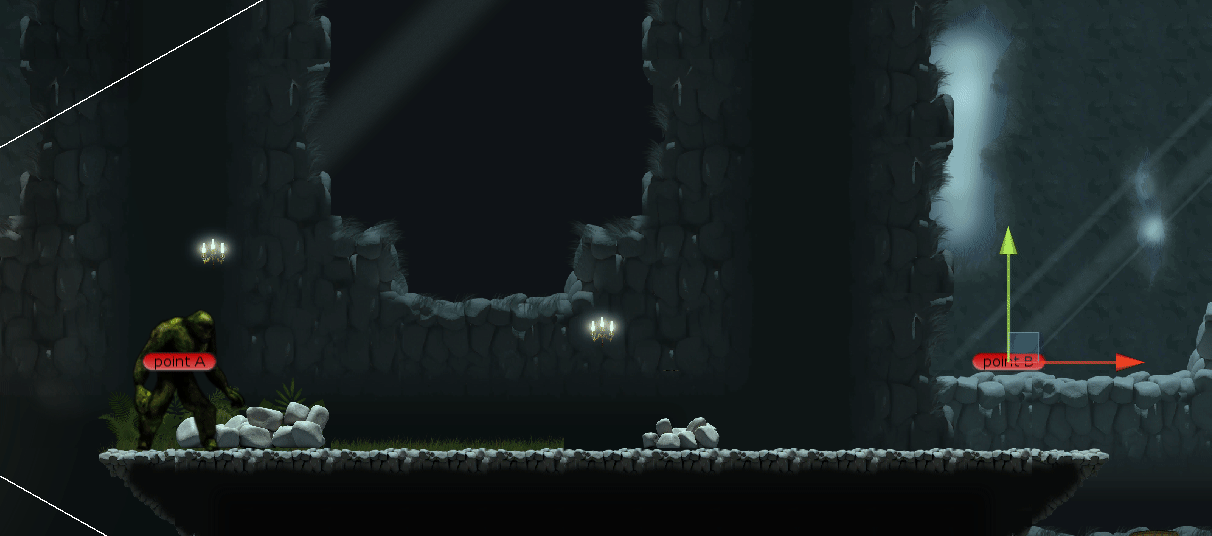## Exception handling : 3 ways illustrated## Annamariya Tharayil

Software Engineer. Find me @ www.linkedin.com/in/annamariya-jt

## [LeetCode]#2078. Two Furthest Houses With Different Colors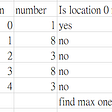## 3 Reasons For Choosing Python as Your First Programming Language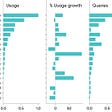## Number of Good Pairs — Day 101(Python)## Implementing Linked Lists in Python